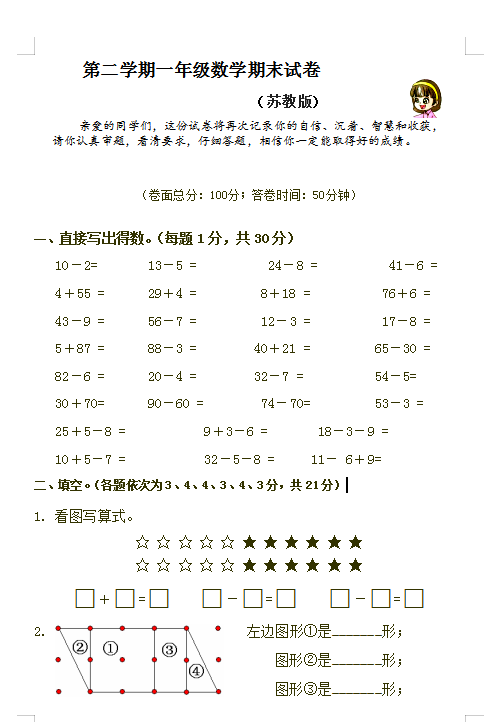苏教版

（卷面总分：100分；答卷时间：50分钟）

10－2=       13－5 =          24－8 =          41－6 =

4＋55 =  29＋4 =         8＋18 =          76＋6 =

43－9 =     56－7 =      12－3 =     17－8 =